• (一)、它是数字滤波器的频率响应（包括幅频...[h,w] = freqz(b,a,n) [h,w] = freqz(sos,n) [h,w] = freqz(d,n) [h,w] = freqz(___,n,'whole') [h,f] = freqz(___,n,fs) [h,f] = freqz(___,n,'whole',fs) h =...
  (一)、它是数字滤波器的频率响应（包括幅频响应和相频响应）

（二）、主要的形式：

[h,w] = freqz(b,a,n)
[h,w] = freqz(sos,n)
[h,w] = freqz(d,n)
[h,w] = freqz(___,n,'whole')
[h,f] = freqz(___,n,fs)
[h,f] = freqz(___,n,'whole',fs)
h = freqz(___,w)
h = freqz(___,f,fs)
freqz(___)
（三）、解释：

[h，w] = freqz（b，a，n）返回数字点滤波器的n点频率响应向量h和相应的角频率向量w，其中数字和分母多项式系数分别存储在b和a中。
[h，w] = freqz（sos，n）返回与二阶部分矩阵sos相对应的n点复频响应。
[h，w] = freqz（d，n）返回数字滤波器d的n点复频响应。注意：当知道滤波器的N个抽头系数之后，可以用这个形式来求滤波器的幅频和相频响应，n如果不指定其默认值为512，也就是返回值h，w的长度都是512点的
[h，w] = freqz（___，n，'whole'）返回整个单位圆周围n个采样点的频率响应。
[h，f] = freqz（___，n，fs）返回给定数字分子和分母多项式系数分别存储在b和a中的数字滤波器的频率响应矢量h和相应的物理频率矢量f采样率fs。
[h，f] = freqz（___，n，'whole'，fs）返回介于0和fs之间的n个点处的频率。
h = freqz（___，w）以w中提供的归一化频率返回频率响应矢量h。
h = freqz（___，f，fs）以f中提供的物理频率返回频率响应矢量h。
没有输出参数的freqz（___）绘制滤波器的频率响应。
注意：如果freqz的输入是单精度的，则频率响应是使用单精度算法计算的。输出h是单精度
（四）、输入输出相关参数解释

输入：
b，a：传递函数的系数向量
传递函数系数，指定为矢量。 用b和a表示传递函数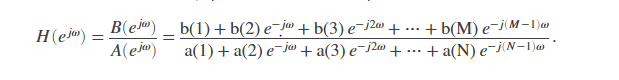例如：
b = [1 3 3 1] / 6和a = [3 0 1 0] / 3指定具有标准化3-dB频率0.5πrad /样本的三阶巴特沃斯滤波器。
数据类型：double | 单
复数支持：是

n —评估点数
512（默认）| 正整数标量
指定为不小于2的正整数标量的评估点数。如果不存在n，则默认为512。为获得最佳结果，请将n设置为大于过滤器阶数的值。

数据类型：双精度

sos —二阶截面系数矩阵
二阶截面系数，指定为矩阵。
sos是一个K×6矩阵，其中部分数K必须大于或等于2。如果部分数小于2，freqz会将输入视为分子向量。 sos的每一行都对应一个二阶（双二阶）滤波器的系数。 sos的第i行对应于[bi（1）bi（2）bi（3）ai（1）ai（2）ai（3）]。

示例：s = [2 4 2 6 0 2; 3 3 0 6 0 0]指定具有标准化3-dB频率0.5πrad /样本的三阶巴特沃斯滤波器。

数据类型：double | 单
复数支持：是

d-数字滤波器
digitalFilter对象
数字滤波器，指定为digitalFilter对象。 使用designfilt根据频率响应规范生成数字滤波器。

示例：d = designfilt（'lowpassiir'，'FilterOrder'，3，'HalfPowerFrequency'，0.5）指定具有标准化3-dB频率0.5πrad /样本的三阶巴特沃斯滤波器。

fs —采样率
正标量
采样率，指定为正标量。 当时间单位为秒时，fs以赫兹表示。

数据类型：双精度

w —角频率
向量
角频率，指定为向量，以弧度/样本表示。 w必须至少包含两个元素。 w =π对应于奈奎斯特频率。

f —频率
向量
频率，指定为向量。 f必须至少包含两个元素。 当时间单位为秒时，f以赫兹表示。

数据类型：双精度

输出：
h-频率响应向量
频率响应，以向量形式返回。如果指定n，则h的长度为n。如果您未指定n或将n指定为空向量，则h的长度为512。
w —角频率向量
角频率，作为矢量返回。 w的取值范围是0到π。如果在输入中指定“ whole”，则w中的值范围为0到2π。如果指定n，则w的长度为n。如果您未指定n或将n指定为空向量，则w的长度为512。
f —频率向量
频率，作为以赫兹表示的向量返回。 f的取值范围是0到fs / 2 Hz。如果在输入中指定“ whole”，则f中的值范围为0到fs Hz。如果指定n，则f的长度为n。如果您未指定n或将n指定为空向量，则f的长度为512。
算法
数字滤波器的频率响应可以解释为在z =ejω处评估的传递函数。
freqz从您指定的（实数或复数）分子和分母多项式确定传递函数，并返回数字滤波器的复数频率响应H（ejω）。在使用的语法确定的采样点上评估频率响应。
当您不提供频率向量作为输入参数时，freqz通常使用FFT算法来计算频率响应。它将频率响应计算为变换后的分子和分母系数的比率，并用零填充到所需的长度。
当您确实提供了一个频率向量作为输入参数时，freqz使用霍纳的嵌套多项式求值方法对每个频率点处的多项式求值，将分子响应除以分母响应。

（五）使用举例：

计算并显示由以下传递函数描述的三阶IIR低通滤波器的幅度响应：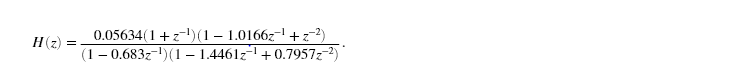将分子和分母表示为多项式卷积。 在整个单位圆上的2001个点处找到频率响应。
b0 = 0.05634;
b1 = [1  1];
b2 = [1 -1.0166 1];
a1 = [1 -0.683];
a2 = [1 -1.4461 0.7957];

b = b0*conv(b1,b2);
a = conv(a1,a2);

[h,w] = freqz(b,a,'whole',2001);

plot(w/pi,20*log10(abs(h)))
ax = gca;
ax.YLim = [-100 20];
ax.XTick = 0:.5:2;
xlabel('Normalized Frequency (\times\pi rad/sample)')
ylabel('Magnitude (dB)')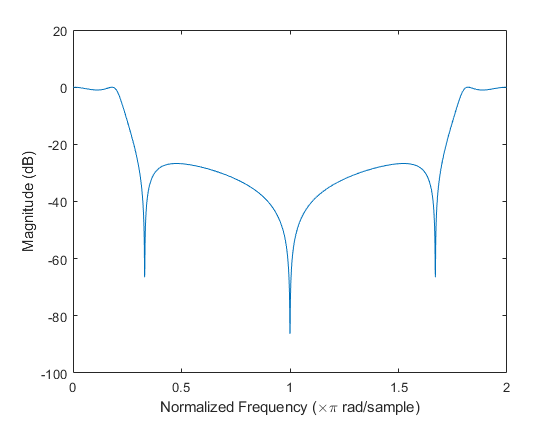使用的Kaiser窗口设计80阶FIR低通滤波器。 指定归一化截止频率rad / sample。
显示滤波器的幅度和相位响应。
b = fir1(80,0.5,kaiser(81,8));
freqz(b,1)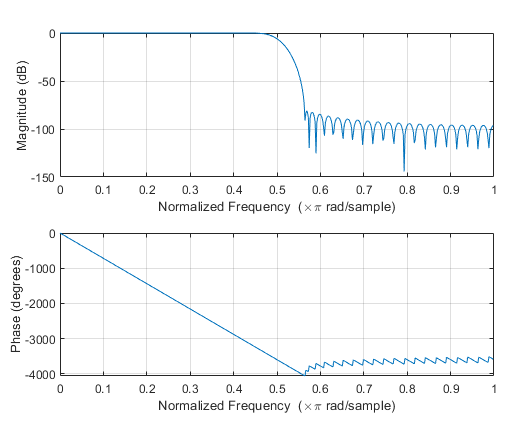用designfilt设计相同的滤波器，用fdatool绘制它的幅频和相频响应：

d = designfilt('lowpassfir','FilterOrder',80, ...
'CutoffFrequency',0.5,'Window',{'kaiser',8});
freqz(d)



展开全文• MATLAB提供了专门用于求离散系统频响特性的函数freqz()，调用freqz()的格式有以下两种：l [H,w]=freqz(B,A,N) B和A分别为离散系统的系统函数分子、分母多项式的系数向量，N为正整数，返回量H则包含了离散系统频响 在...
MATLAB提供了专门用于求离散系统频响特性的函数freqz()，调用freqz()的格式有以下两种：l        [H,w]=freqz(B,A,N)    B和A分别为离散系统的系统函数分子、分母多项式的系数向量，N为正整数，返回量H则包含了离散系统频响 在 0——pi范围内N个频率等分点的值，向量w则包含 范围内N个频率等分点。调用中若N默认，默认值为512。l        [H,w]=freqz(B,A,N,’whole’)该调用格式将计算离散系统在0—pi范内的N个频率等分店的频率响应的值。因此，可以先调用freqz()函数计算系统的频率响应，然后利用abs()和angle()函数及plot()函数，即可绘制出系统在 或 范围内的频响曲线。
展开全文• MATLAB 总结;2;3;4;5;6;7;8;10;10;10
• ## 详解MATLAB之freqz()函数

万次阅读 多人点赞 2018-03-05 12:54:50
一 函数调用介绍MATLAB提供了专门用于求离散系统频响特性的函数freqz()，调用freqz()的格式有以下几种：(1)[H,w]=freqz(B,A,N); %N默认值为512(2)[H,w]=freqz(B,A,N,'whole');(3)[H,w]=freqz(B,A,[自定义区间]);上式...
一 函数调用介绍MATLAB提供了专门用于求离散系统频响特性的函数freqz()，调用freqz()的格式有以下几种：(1)[H,w]=freqz(B,A,N);  %N默认值为512(2)[H,w]=freqz(B,A,N,'whole');(3)[H,w]=freqz(B,A,[自定义区间]);上式中B和A分别对应离散系统的系统函数H(z)的分子、分母多项式的系数向量：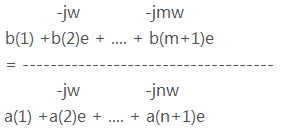B=[b1,b2,...];    A=[a1,a2,...];返回量H则包含了离散系统对应区间内N（N为正整数）个频率等分点的频率响应，w为N个频率等分点的值。(1)式调用默认区间 0：pi(2)式调用主值区间 -pi：pi(3)式自定义区间，如[0:2*pi/n:2*pi]最后利用abs()和angle()函数及plot()函数，即可绘制出系统在频率区间的频响曲线。二 示例绘制如下系统的频响曲线： H(z)=(1-0.5z^-1)MATLAB命令如下：B=[1 -0.5]; A =;[H,w]=freqz(B,A,[0:2*pi/400:2*pi]);Hf=abs(H);  %取幅度值实部Hx=angle(H);  %取相位值对应相位角clffigure(1)plot(w,20*log(Hf))  %幅值变换为分贝单位title('离散系统幅频特性曲线')figure(2)plot(w,Hx)title('离散系统相频特性曲线')
展开全文• 本文引用自乱七八糟《MATLABfreqz函数的用法》    FREQZ 是计算数字滤波器的频率响应的函数  [H,W] = FREQZ(B,A,N)returns the N-point complex frequency response  vector H and the N-point frequency ...


本文引用自乱七八糟《MATLAB中freqz函数的用法》

FREQZ 是计算数字滤波器的频率响应的函数
[H,W] = FREQZ(B,A,N)returns the N-point complex frequency response
vector H and the N-point frequency vector W in radians/sample of
the filter:
函数的输出：a.滤波器的频率响应H（N点）  b.频率向量W(N点，且单位为弧度)
其中，滤波器形式如下：given numerator and denominator coefficients in vectors B and A. The
frequency response is evaluated at N points equally spaced around the
upper half of the unit circle. If N isn't specified, it defaults to    512.
滤波器的系数：
分子为B，分母为A
频率向量W，是均匀分布在滤波器的上半区，即：0：pi，这些点上的频率响应都将通过此函数计算出来。
举例:
假设滤波器的系数A=1，B=【1 1/2 1/3 1/4】;通过FREQZ函数便可以得到此滤波器的频率特性
其中，需要考察的是H和W的长度，看是否符合我们设置的N。[H,W] = FREQZ(B,A,N,'whole')uses N points around the whole unit circle.
函数给出了滤波器的双边频率特性，是N点的
H = FREQZ(B,A,W)returns the frequency response at frequencies
designated in vector W, in radians/sample (normally between 0 and pi).
函数的输出依然是滤波器的频率响应H，只不过这个H是限定了范围的，不再是全频率（0：pi）上的了，这个范围由W来指定，单位是弧度。
举例：W=【0.5pi----pi】,下图中，红线部分便是此函数的输出，可见，只描述了【0.5pi----pi】之间的频率响应。
[H,F] = FREQZ(B,A,N,Fs) and [H,F] = FREQZ(B,A,N,'whole',Fs)return
frequency vector F (in Hz), where Fs is the sampling frequency (in Hz).
注意下图的横坐标与程序中fs的关系

H = FREQZ(B,A,F,Fs)returns the complex frequency response at the
frequencies designated in vector F (in Hz), where Fs is the sampling
frequency (in Hz).
FREQZ(B,A,...)with no output arguments plots the magnitude and
unwrapped phase of the filter in the current figure window.


展开全文vector filter output
• >> help freqz freqz - Frequency response of filter  滤波器的频率响应 ... This MATLAB function returns the frequency response h and the corresponding  frequencies w at which the filter re
• freqz函数计算线性系统的频率响应,包括幅频响应和相频响应,基本输入为线性系统的AMMA模型系数向量,一个典型的AMMA模型为 % jw -jw -jmw % jw B(e) b(1) + b(2)e + .... + b(m+1)e % H(e) = ---- = ----
• freqz函数计算线性系统的频率响应,包括幅频响应和相频响应,基本输入为线性系统的AMMA模型系数向量,一个典型的AMMA模型为 %jw-jw-jmw%jwB(e)b(1) + b(2)e + .... + b(m+1)e%H(e) = ---- = ----------...
• 函数法设计FIRDF使用Matlab的fir1（）函数十分方便，DSP课堂上已经讲过，此处不再赘述。关心的是：使用 hn = fir1（M， wc）；...首先上matlab的help查freqz（）函数：大概意思就是说freqz是0~π的n点频率响应，hf...数字信号处理 滤波器设计
• freqz Frequency response of digital filter Syntax [h,w] = freqz(b,a,n) [h,w] = freqz(d,n) [h,w] = freqz(___,n,'whole') freqz(___) [h,f] = freqz(___,n,fs) [h,f] = freqz(___,n,'whole',fs) h = f...
• ## matlabfreqz函数使用

千次阅读 2016-11-08 09:25:47
Matlab freqz函数使用 (2012-10-22 13:42:03) 转载▼ 标签： 杂谈 freqz函数计算线性系统的频率响应,包括幅频响应和相频响应,基本输入为线性系统的AMMA模型系数向量,一个典型的AMMA...
• ## matlabFREQZ函数

千次阅读 2016-08-09 10:14:01
H = FREQZ(B,A,W)returns the frequency response at frequencies   designated in vector W, in radians/sample (normally between 0 and pi).   函数的输出依然是滤波器的频率响应H，只不过这个H是限定了...
• ## matlab的freqz

万次阅读 2006-11-01 19:58:00
MATLAB提供了专门用于求离散系统频响特性的函数freqz()，调用freqz()的格式有以下两种：l [H,w]=freqz(B,A,N) B和A分别为离散系统的系统函数分子、分母多项式的系数向量，N为正整数，返回量H则包含了离散系统频响 ...plot
• 对于一个变量a，matlab中定义需要这样 syms a； 定义之后就可以写由变量组成的式子，比如 c=(1+...MATLAB提供了专门用于求离散系统频响特性的函数freqz()，调用freqz()的格式有以下两种： l[H,w]=freqz(B,A,N) ...
• MATLAB提供了专门用于求离散系统频响特性的函数freqz()，调用freqz()的格式有以下两种： l[H,w]=freqz(B,A,N) B和A分别为离散系统的系统函数分子、分母多项式的系数向量，N为正整数，返回量H则包含了离散系统...
• MATLAB提供了专门用于求离散系统频响特性的函数freqz()，调用freqz()的格式有以下两种：  [H,w]=freqz(B,A,N)    B和A分别为离散系统的系统函数分子、分母多项式的系数向量，N为正整数，返回量H则包含了...
• 关于滤波器设计实现的Matlab 函数 分类 函数名 功能说明 abs 求模值 angle 求相角 freqs 模拟滤波器的频率响应 滤波器分析 freqz 数字滤波器的频率响应 求幅频相频响应 grpdelay 群延迟 impz 脉冲响应 离散的 zplane...
• matlab中的freqz()函数可以用来求得系统的频率响应。但同时freqz()函数还有另外一个功能，那就是求得序列的离散时间傅里叶变换(DTFT)。 $[h, f] = freqz(b,a)$ 其中 b,a分别是系统传递函数的分子和分母的系数，h是...数字信号处理
•  B和A分别为离散系统的系统函数分子、分母多项式的系数向量，N为正整数，返回量H则包含了离散系统频响 在 0——pi范围内N个频率等分点的值，向量w则包含 范围内N个频率等分点。调用中若N默认，默认值为512。 ...plot
•数字信号处理
• 函数一：[H,w]=freqz(B,A,N)MATLAB提供了专门用于求离散系统频响特性的函数freqz()，调用freqz()的格式有以下两种：l[H,w]=freqz(B,A,N)B和A分别为离散系统的系统函数分子、分母多项式的系数向量，N为正整数，返回量...
• 新版的matlab中取消了freqz_m函数，如果以前编的程序中有用到这个函数，可以拿来直接用
• %使用窗函数设计低通滤波器 %经过计算N=40使用汉宁窗时有最小的长度 %author clc; clear all; N=40; wc=0.5; w=hanning(N+1; b = fir1(N,0.5,w; [h,omega] = freqz(b,1; plot(omega/pi,20*log10(abs(h;grid; ylim([-...
• freqz函数函数可以求取离散系统的频响特性 画出幅频特性曲线和相频特性曲线 unwrap函数介绍 此函数可以解卷绕 ，可以防止相位跳变 unwrap(pha,tol,dim)； pha 可为数列或矩阵，tol 为判断的阈值，dim 参数可以...iir滤波器
• matlab 数字信号处理函数 matlab实现数字信号处理的一些经典理论 内涵： 滤波器的设计，模拟与数字 采样定律 Z变换与s域映射 卷积原因 截断效应 各种变换 如：DFS DFT IDFT 具体的如下： % 离散信号和系统 % conv_m...# matlab函数freqzmatlab 订阅